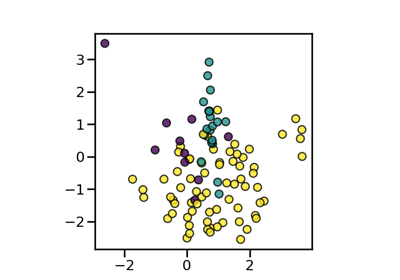# SMOTEENN#

class imblearn.combine.SMOTEENN(*, sampling_strategy='auto', random_state=None, smote=None, enn=None, n_jobs=None)[source]#

Over-sampling using SMOTE and cleaning using ENN.

Combine over- and under-sampling using SMOTE and Edited Nearest Neighbours.

Read more in the User Guide.

Parameters
sampling_strategyfloat, str, dict or callable, default=’auto’

Sampling information to resample the data set.

• When float, it corresponds to the desired ratio of the number of samples in the minority class over the number of samples in the majority class after resampling. Therefore, the ratio is expressed as $$\alpha_{os} = N_{rm} / N_{M}$$ where $$N_{rm}$$ is the number of samples in the minority class after resampling and $$N_{M}$$ is the number of samples in the majority class.

Warning

float is only available for binary classification. An error is raised for multi-class classification.

• When str, specify the class targeted by the resampling. The number of samples in the different classes will be equalized. Possible choices are:

'minority': resample only the minority class;

'not minority': resample all classes but the minority class;

'not majority': resample all classes but the majority class;

'all': resample all classes;

'auto': equivalent to 'not majority'.

• When dict, the keys correspond to the targeted classes. The values correspond to the desired number of samples for each targeted class.

• When callable, function taking y and returns a dict. The keys correspond to the targeted classes. The values correspond to the desired number of samples for each class.

random_stateint, RandomState instance, default=None

Control the randomization of the algorithm.

• If int, random_state is the seed used by the random number generator;

• If RandomState instance, random_state is the random number generator;

• If None, the random number generator is the RandomState instance used by np.random.

smotesampler object, default=None

The SMOTE object to use. If not given, a SMOTE object with default parameters will be given.

ennsampler object, default=None

The EditedNearestNeighbours object to use. If not given, a EditedNearestNeighbours object with sampling strategy=’all’ will be given.

n_jobsint, default=None

Number of CPU cores used during the cross-validation loop. None means 1 unless in a joblib.parallel_backend context. -1 means using all processors. See Glossary for more details.

Attributes
sampling_strategy_dict

Dictionary containing the information to sample the dataset. The keys corresponds to the class labels from which to sample and the values are the number of samples to sample.

smote_sampler object

The validated SMOTE instance.

enn_sampler object

The validated EditedNearestNeighbours instance.

n_features_in_int

Number of features in the input dataset.

New in version 0.9.

feature_names_in_ndarray of shape (n_features_in_,)

Names of features seen during fit. Defined only when X has feature names that are all strings.

New in version 0.10.

SMOTETomek

Over-sample using SMOTE followed by under-sampling removing the Tomek’s links.

Notes

The method is presented in .

Supports multi-class resampling. Refer to SMOTE and ENN regarding the scheme which used.

References

1

G. Batista, R. C. Prati, M. C. Monard. “A study of the behavior of several methods for balancing machine learning training data,” ACM Sigkdd Explorations Newsletter 6 (1), 20-29, 2004.

Examples

>>> from collections import Counter
>>> from sklearn.datasets import make_classification
>>> from imblearn.combine import SMOTEENN
>>> X, y = make_classification(n_classes=2, class_sep=2,
... weights=[0.1, 0.9], n_informative=3, n_redundant=1, flip_y=0,
... n_features=20, n_clusters_per_class=1, n_samples=1000, random_state=10)
>>> print('Original dataset shape %s' % Counter(y))
Original dataset shape Counter({1: 900, 0: 100})
>>> sme = SMOTEENN(random_state=42)
>>> X_res, y_res = sme.fit_resample(X, y)
>>> print('Resampled dataset shape %s' % Counter(y_res))
Resampled dataset shape Counter({0: 900, 1: 881})


Methods

 fit(X, y) Check inputs and statistics of the sampler. fit_resample(X, y) Resample the dataset. get_feature_names_out([input_features]) Get output feature names for transformation. get_params([deep]) Get parameters for this estimator. set_params(**params) Set the parameters of this estimator.
fit(X, y)[source]#

Check inputs and statistics of the sampler.

You should use fit_resample in all cases.

Parameters
X{array-like, dataframe, sparse matrix} of shape (n_samples, n_features)

Data array.

yarray-like of shape (n_samples,)

Target array.

Returns
selfobject

Return the instance itself.

fit_resample(X, y)[source]#

Resample the dataset.

Parameters
X{array-like, dataframe, sparse matrix} of shape (n_samples, n_features)

Matrix containing the data which have to be sampled.

yarray-like of shape (n_samples,)

Corresponding label for each sample in X.

Returns
X_resampled{array-like, dataframe, sparse matrix} of shape (n_samples_new, n_features)

The array containing the resampled data.

y_resampledarray-like of shape (n_samples_new,)

The corresponding label of X_resampled.

get_feature_names_out(input_features=None)[source]#

Get output feature names for transformation.

Parameters
input_featuresarray-like of str or None, default=None

Input features.

• If input_features is None, then feature_names_in_ is used as feature names in. If feature_names_in_ is not defined, then the following input feature names are generated: ["x0", "x1", ..., "x(n_features_in_ - 1)"].

• If input_features is an array-like, then input_features must match feature_names_in_ if feature_names_in_ is defined.

Returns
feature_names_outndarray of str objects

Same as input features.

get_params(deep=True)[source]#

Get parameters for this estimator.

Parameters
deepbool, default=True

If True, will return the parameters for this estimator and contained subobjects that are estimators.

Returns
paramsdict

Parameter names mapped to their values.

set_params(**params)[source]#

Set the parameters of this estimator.

The method works on simple estimators as well as on nested objects (such as Pipeline). The latter have parameters of the form <component>__<parameter> so that it’s possible to update each component of a nested object.

Parameters
**paramsdict

Estimator parameters.

Returns
selfestimator instance

Estimator instance.

## Examples using imblearn.combine.SMOTEENN#Compare sampler combining over- and under-sampling

Compare sampler combining over- and under-sampling